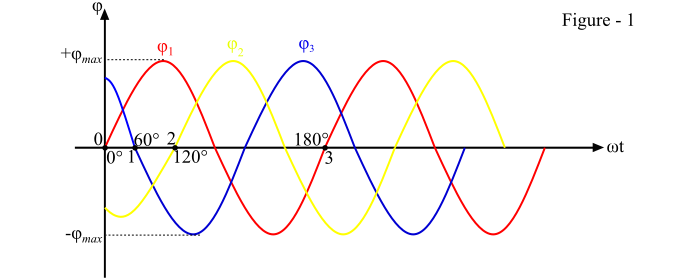# Rotating Magnetic Field in a 3-Phase Induction Motor

Consider three identical coils located on axes physically at 120° in space and each coil is supplied from one phase of a balanced 3-phase supply. Thus, each coil will produce an alternating flux along its own axis. Let the instantaneous values of the fluxes are given by,

$$\mathrm{\varphi_{1} = \varphi_{m} sin \omega t … (1)}$$

$$\mathrm{\varphi_{2} = \varphi_{m} sin(\omega t − 120°) … (2)}$$

$$\mathrm{\varphi_{3} = \varphi_{m} sin(\omega t + 120°) … (3)}$$The waveforms of the fluxes produced by the three coils are shown in Figure-1. The resultant flux (ϕr) at any instant is equal to the phasor sum of the fluxes due to three phases. To determine the values of resultant flux (ϕr), we consider four point viz. 0, 1, 2 and 3 which are 60° apart.

## Case I – When ωt = 0°

This instant is corresponds to the point 0 in the waveform. Putting ωt = 0° in the equations (1), (2) and (3), we get,

$$\mathrm{\varphi_{1} = \varphi_{m} sin 0° = 0}$$

$$\mathrm{\varphi_{2} = \varphi_{m} sin(0° − 120°) = −\frac{\sqrt{3}}{2}\varphi_{m}}$$

$$\mathrm{\varphi_{3} = \varphi_{m} sin(0° + 120°) =\frac{\sqrt{3}}{2}\varphi_{m}}$$Refer Figure-2, the phasor for ϕ2 is shown along OY and the phasor for ϕ3 is shown along OB. Thus the resultant flux ϕr is the phasor sum of OY and OB which is shown along OA. The magnitude of the resultant flux is given by,

$$\mathrm{\varphi_{r} = 𝑂𝐴 = 2𝑂𝐸 = 2 \:𝑂𝐵\: cos 30° = 2 \times\frac{\sqrt{3}}{2}\varphi_{m} \times\frac{\sqrt{3}}{2}=\frac{3}{2}\varphi_{m}}$$

## Case II – When ωt = 60°

This instant is corresponds to point 1. Putting ωt = 60° in the equations (1), (2) and (3), we get,

$$\mathrm{\varphi_{1} = \varphi_{m} sin 60° =frac{\sqrt{3}}{2}\varphi_{m}}$$

$$\mathrm{\varphi_{2} = \varphi_{m} sin(60° − 120°) = −frac{\sqrt{3}}{2}\varphi_{m}}$$

$$\mathrm{\varphi_{3} = \varphi_{m} sin(60° + 120°) = 0}$$

The phasors ϕ1, ϕ2 and ϕr are shown in Figure-3. The value of resultant flux is given by,

$$\mathrm{\varphi_{r} = 𝑂𝐴 = 2\: 𝑂𝑅 \:cos 30° = 2 \times\frac{\sqrt{3}}{2}\varphi_{m} \times\frac{\sqrt{3}}{2}=\frac{3}{2}\varphi_{m}}$$

Hence, it can be seen that the resultant flux is again $(\frac{3}{2} \varphi_m)$ but has rotated through an angle of 60° in the clockwise direction.

## Case III - When ωt = 120°

This instant is corresponds to point 2. Putting ωt = 120° in the equations (1), (2) and (3), we get,

$$\mathrm{\varphi_{1} = \varphi_{m} sin 120° =\frac{\sqrt{3}}{2}\varphi_{m}}$$

$$\mathrm{\varphi_{2} = \varphi_{m} sin(120° − 120°) = 0}$$

$$\mathrm{\varphi_{3} = \varphi_{m} sin(120° + 120°) = −\frac{\sqrt{3}}{2}\varphi_{m}}$$

Thus, the resultant flux is given by,

$$\mathrm{\varphi_{r} = 𝑂𝐴 = 2\:𝑂𝑅\:cos 30° = 2 \times \frac{\sqrt{3}}{2}\varphi_{m} \times\frac{\sqrt{3}}{2}=\frac{3}{2}\varphi_{m}}$$

Hence, the resultant flux is again $(\frac{3}{2} \varphi_m)$ obtained but has further rotated by an angle of 60° form point 1 in the clockwise direction (see Figure-4).

## Case IV – When ωt = 180°

This instant is corresponds to point 3. Putting ωt = 180° in the equations (1), (2) and (3), we get,

$$\mathrm{\varphi_{1} = \varphi_{m} sin 180° = 0}$$

$$\mathrm{\varphi_{2} = \varphi_{m} sin(180° − 120°) =\frac{\sqrt{3}}{2}\varphi_{m}}$$

$$\mathrm{\varphi_{3} = \varphi_{m} sin(180° + 120°) = −\frac{\sqrt{3}}{2}\varphi_{m}}$$

Thus, the resultant flux is given by,

$$\mathrm{\varphi_{r} = 𝑂𝐴 = 2\:𝑂𝑌\:cos 30° = 2 \times\frac{\sqrt{3}}{2}\varphi_{m} \times\frac{\sqrt{3}}{2}=\frac{3}{2}\varphi_{m}}$$

Again, the resultant flux is equal to $(\frac{3}{2} \varphi_m)$ but has further rotated by an angle of 60° form the point 2 in the clockwise direction (see Figure-5).

From the above discussion, it is clear that a three phase balanced supply produces a rotating magnetic field.